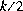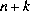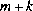Contents | Prev | Next | Index

# 22.12 The Class `java.io.LineNumberInputStream`

A `LineNumberInputStream` adds functionality to another input stream, namely the ability to count lines. When the `LineNumberInputStream` is created, the line number counter is set to zero. As bytes from the stream are read or skipped, the counter is incremented whenever a line terminator (`\n`, `\r`, or `\r\n`) is encountered. Such line terminators are also converted to a single `'\n'` character. The method `getLineNumber` returns the current value of the counter, and the method `setLineNumber` sets the counter to a given integer value. If the contained input stream supports the `mark` operation, then so does the `LineNumberInputStream`; the `mark` operation remembers the line number counter and the `reset` operation sets the counter to the value remembered by the `mark` operation.

```public class `LineNumberInputStream` extends FilterInputStream {
public `LineNumberInputStream`(InputStream in);
public int `read`() throws IOException;
public int `read`(byte[] b)
throws IOException, NullPointerException;
public int `read`(byte[] b, int off, int len)
throws IOException, NullPointerException,
IndexOutOfBoundsException;
public long `skip`(long n) throws IOException;
public int `available`() throws IOException;
public void `mark`(int readlimit);
public void `reset`() throws IOException;
public int `getLineNumber`();
public void `setLineNumber`(int lineNumber);
}
```

22.12.1 `public LineNumberInputStream(InputStream in)`

This constructor initializes a newly created `LineNumberInputStream` by saving its argument, the input stream `in`, for later use.

22.12.2 `public int read() throws IOException`

See the general contract of the `read` method of `InputStream` (§22.3.1).

As bytes are read from the contained input stream, line terminators are recognized and counted. For each line terminator recognized in the contained input stream, a single character `'\n'` is returned.

Overrides the `read` method of `FilterInputStream` (§22.9.3).

22.12.3 `public int read(byte[] b)throws IOException, NullPointerException`

See the general contract of the `read` method of `InputStream` (§22.3.2).

As bytes are read from the contained input stream, line terminators are recognized and counted. For each line terminator recognized in the contained input stream, a single character `'\n'` is returned.

Overrides the `read` method of `FilterInputStream` (§22.9.4).

22.12.4 `public int read(byte[] b, int off, int len)throws IOException, NullPointerException, IndexOutOfBoundsException`

See the general contract of the `read` method of `InputStream` (§22.3.3).

As bytes are read from the contained input stream, line terminators are recognized and counted. For each line terminator recognized in the contained input stream, a single character `'\n'` is returned.

Overrides the `read` method of `FilterInputStream` (§22.9.5).

22.12.5 `public long skip(long n) throws IOException`

See the general contract of the `skip` method of `InputStream` (§22.3.4).

As bytes are read from the contained input stream, line terminators are recognized and counted. Each line terminator recognized in the contained input stream is considered to be a single byte skipped, even if it is the sequence `\r\n`.

Overrides the `skip` method of `FilterInputStream` (§22.9.6).

22.12.6 `public int available() throws IOException`

See the general contract of the `available` method of `InputStream` (§22.3.5).

Note that if the contained input stream is able to supply k input characters without blocking, the `LineNumberInputStream` can guarantee only to providecharacters without blocking, because the k characters from the contained input stream might consist of`\r\n` pairs, which will be converted to just`'\n'` characters.

Overrides the `available` method of `FilterInputStream` (§22.9.7).

22.12.7 `public void mark(int readlimit)`

See the general contract of the `mark` method of `InputStream` (§22.3.7).

Marking a point in the input stream remembers the current line number as it would be returned by `getLineNumber` (§22.12.9).

Overrides the `mark` method of `FilterInputStream` (§22.9.9).

22.12.8 `public void reset() throws IOException`

See the general contract of the `reset` method of `InputStream` (§22.3.8).

Resetting the input stream to a previous point also resets the line number to the value it had at the marked point.

Overrides the `reset` method of `FilterInputStream` (§22.9.10).

22.12.9 `public int getLineNumber()`

The current line number is returned. This quantity depends on k, the number of line terminators encountered since the most recent occurrence of one of the following three kinds of events:

• If a call to the `setLineNumber` method was most recent, let n be the argument that was given to `setLineNumber`; then the current line number is.
• If a call to the `reset` method was most recent, let m be the line number that had been remembered by `mark`; then the current line number is.
• If creation of the `LineNumberInputStream` was most recent (that is, neither of the other kinds of event have occurred), then the current line number is k.
These rules imply that the current line number is `0` as the characters of the first line are read, and becomes `1` after the line terminator for the first line has been read.

22.12.10 `public void setLineNumber(int lineNumber)`

The current line number is set equal to the argument.

Java Language Specification (HTML generated by Suzette Pelouch on February 24, 1998)